# Overall Class Structure ( proposed)

Review Previous learning and intro to current class activities or new learning 15 - 20 mins

Independent work 20-30

Teacher one to one check progress ( we can swap this around )

• Arin -   11:30 - 11:35
• Shiva -   11:35 - 11:40
• Girwin -   11:40 - 11:45
• Sang -   11:45- 11:50
• Joshu -   11:50 - 11:55
• Irfan -   11:55 - 12:00

12:00 Get together - Set final Activity / Home work / Grade Book

12:20 Get together - Completed may leave else stay for further guidance on activity / topic

# Lesson Block 1 26th August 2021

### Review 10mins

4.3.1 State the fundamental operations of a computer.

4.3.2 Distinguish between fundamental and compound operations of a computer

Operations on a collection ?

Operations on a stack ?

Operations on a array?

Operations on a queue?

### 30 mins -  15 Minutes  10 Mins Activity 5 Discussion

Q8 [3marks]

List the output from the following input ( 10mins )

2,6,8,9,12,15,20

Loop Count from 0 to 7

INPUT Number

If NUMBER div 2 = NUMBER /2  then

if NUMBER div 3 = NUMBER/ 3 then

OUTPUT Number

Programming Activity (15 mins)

Create  a working program based on the Pseudocode  in Python or language of your choice.

# Lesson Block 2 02 Sep 2021

### MOD Operator

The modulus operator - or more precisely, the modulo operation - is a way to determine the remainder of a division operation. Instead of returning the result of the division, the modulo operation returns the whole number remainder.

Question:
What is 5 divided by 2?

## Dividing Numbers

ivision is one of the four main operations in math. When you divide a number, you are basically just splitting it into even groups. For example, 24 divided into 3 even groups would mean you have 8 groups (24 / 3 = 8). Sometimes a number divides evenly, but other times you have numbers left over. These numbers are called the remainder.

The number 5 divided by 2 is 2 with a remainder of 1 (5 / 2 = 2 R.1). This is also written as 5 /2 = 2.5. When you divide 5 by 2, it does not come out evenly. For example, you can't split five oranges into even groups of two, so you would have two groups of two oranges each, with one orange left over.

** It may be helpful to think back to your early math lessons, before you learned fractions and decimals. Mathematics with whole numbers behaves differently - when dividing numbers that aren’t even multiples, there’s always some amount left over. That remainder is what the modulo operation returns.

Some examples may help illustrate this, as it’s not necessarily intuitive the first time you encounter it:

``5 % 1 = 0 // 5 divided by 1 equals 5, with a remainder of 0 5 % 2 = 1 // 5 divided by 2 equals 2, with a remainder of 1 5 % 3 = 2 // 5 divided by 3 equals 1, with a remainder of 2 5 % 4 = 1 // 5 divided by 4 equals 1, with a remainder of 1 5 % 5 = 0 // 5 divided by 5 equals 1, with a remainder of 0``

What is 100 mod 10 ?

What is 233 mod 10?

what is 2005 mod 100

Remeber ask yourself how many groups of 10's are there what is left over is the MOD

#### Starter Past paper 2015

A sub-program all_even() accepts a positive integer N and outputs true if all digits of N are even, otherwise it outputs false. For example, all_even(246) outputs true and all_even(256) outputs false.

The following algorithm is constructed for the sub-program all_even(N).

EVEN = true

loop while (N > 0) and (EVEN = true)

if (N mod 10)mod 2 = 1 then

EVEN = false

end if

end loop

output EVEN

(a) Explain why this algorithm does not obtain the correct result. 

(b) Outline what should be changed in the algorithm to obtain the correct result. 

### 30 mins (high Level) -  15 Minutes Pseudo  15 Mins Programming Activity

#### For reference Past Paper May 2021

14. The following flowchart is intended to represent an algorithm in which numbers that are input cannot be negative.

The flowchart contains a logic error that will affect the algorithm's functionality14 a (i) Identify the logic error 

14 a (ii) Outline how the error in part Ii) can be corrected 

14 a (ii) Outline how the error in part Ii) can be corrected 

A further change has been requested to enable the calc of the average of the  numbers entered. The avaerage should be output at the end of the program.

Based on flow chart construct an algoroithm using pseudocode. That inlcudes the required changes.  

• Correction of logic error
• Only accept integeres between 0 and 100
• Calc the average of all the numbers entered
• Output of final average

Convert pseudo code into a working program

# Lesson Block  09 Sep 2021

4.2.3 Discuss an algorithm to solve a specific problem.

Students should be expected to discuss the differences between algorithms, including both standard and novel algorithms. For example, discussing the advantages and disadvantages of using a binary search as opposed to a sequential search.

4.1.2  Evaluate whether the order in which activities are undertaken will result in the required outcome

4.1.3  Explain the role of sub-procedures in solving a problem

This means TESTING an algorithm using SAMPLE DATA.For example, the following should add up all positive numbers in an array containing 5 numbers. Test with NUMS = [ 5 , 5 , 0, 2 , 2 ]

Loop C from 0 to 4

SUM = 0

SUM = SUM + NUMS[C]

end loop

OUTPUT SUM

1) The algorithm has 1 issues which is the order of activities, identify the problem it and write correct code in Python

2) The algorithm requirement has changed in that it should accept an array of positive and negative integers, but only output sum the positive integers

3) Is your program well structured? Can it be compartmentalised and reused ? If not rework your program, so it is well structured

4) List 4 advantages of a well structure program.

## How to implement a Queue using a Linked List

We will implement Queue using linked list. Queues maintain two data pointers:

• FRONT: to know first inserted element
• REAR to know last inserted element.
• Queue is  a very simple data structure, you only have to manipulate FRONT and REAR to get Queue property.

### Basic Operation:

Main Queue Operations:
1)EnQueue(): Inserts an element at the rear of the Queue.
2)DeQueue(): Remove and return the front element of the Queue.

Define two Node pointers 'front' and 'rear' and set both to NULL.

Queue is very simple data structure, you only have to manipulate FRONT and REAR to get Queue property.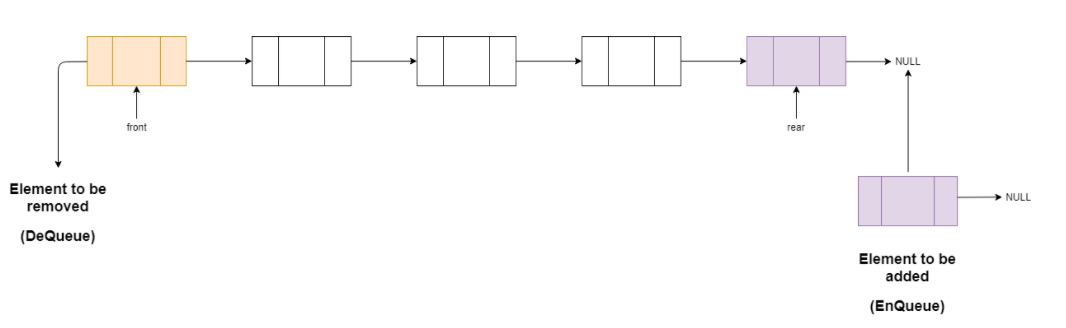``Step 1 : Create a newNode with given value and set 'newNode → next' to NULL. ``Step 2 : Check whether queue is Empty (rear == NULL) ``Step 3 : If it is Empty then, set front = newNode and rear = newNode. ``Step 4 : If it is Not Empty then, set rear → next = newNode and rear = newNode.``

### EnQueue

Inserting an element in Queue.

• Initially, both of our pointers pointing to the front and rear are NULL.
• Now,If We enter the 12 in Queue we will checking weather there is any element present or not. If rear is NULL, We make our front and rear pointers to point our newly inserted Node with having data '12'.
• Now, again if we want to enter new data, Let say 45. We will check for rear NULL or not. Now our rear would be pointing to '12' .So 45 will added next to the rear Node and 45 would be our rear Node.
• Same way if i add 26, it would be added after 45 and rear will point to the Node having data '26'.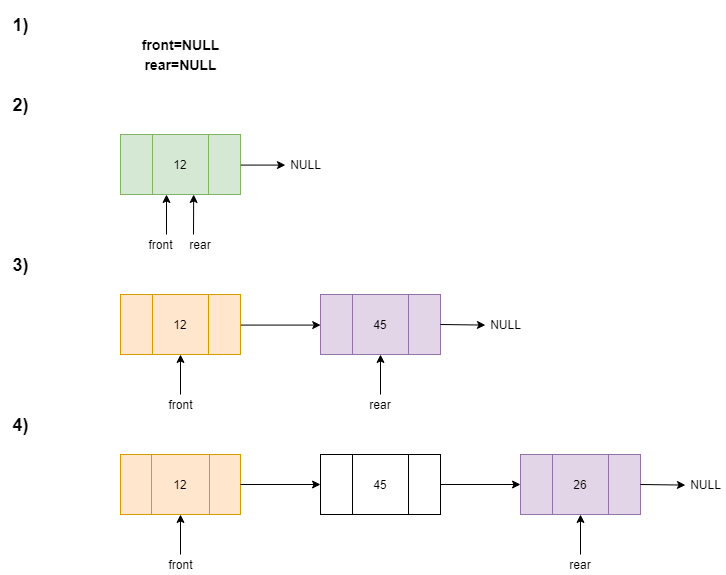``Step 1 : Check whether queue is Empty (front == NULL). ``Step 2 : ``If it is Empty, then display "Queue is Empty!!! Deletion is not possible!!!" and terminate from ``the functionStep 3 : If it is Not Empty then, define a Node pointer 'temp' and set it to 'front'. ``Step 4 : Then set 'front = front → next' and delete 'temp' (free(temp)).``

## Complexity

• Time complexity of DeQueue and EnQueue operation is O(1),Because there is no loop in those operation.
• Time complexity of Display operation is O(n), Since we are traversing to print each n(number of elements) elements.
• Space complexity is O(n), Where n is number of elements.

Explain why a stack is not an appropriate data structure for managing queues.

Draw a diagram or diagrams to show how a stack can be implemented using a linked list.

# Lesson Block  16 Sep 2021

### 4.3.3 Explain the essential features of a computer language.

For example, fixed vocabulary, unambiguous meaning, consistent grammar and syntax

What is the difference between a Syntactical and a Semantic error in a program?

### 4.2.1 Describe the characteristics of standard algorithms on linear arrays

These are: sequential search, binary search, bubble sort, selection sort

### Task Complete part A by 11:30

Work on 13 part a  and share your pseudo code solution on forum link here

### Task Complete part B, C and D by 12:15

Work on 13 part b ,c and d  and share your pseudo code solution on forum link here### BUBBLE SORT### Task Last 20 mins  or homework

The code below is close but will not work, it has a semantic error. Your task is to get the program to working in PYTHON.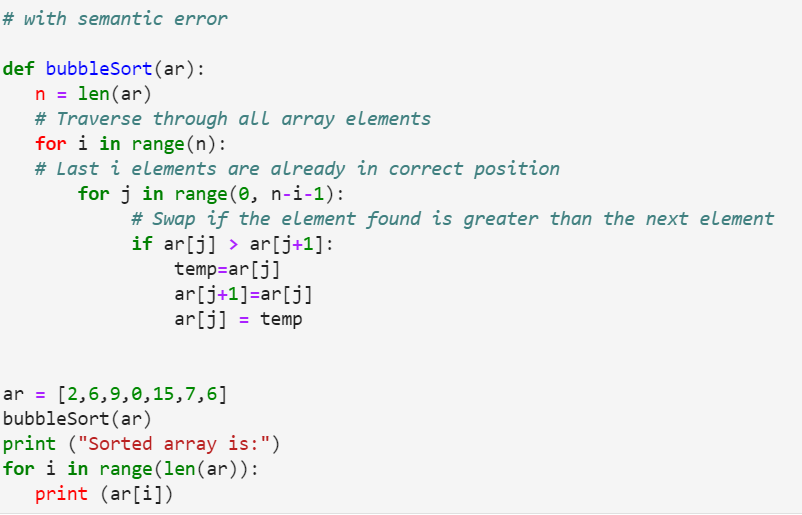## Selection Visual Representation

Given a list of five elements, the following images illustrate how the selection sort algorithm iterates through the values when sorting them.

The following image shows the unsorted listStep 1)The first value 21 is compared with the rest of the values to check if it is the minimum value.3 is the minimum value, so the positions of 21 and 3 are swapped. The values with a green background represent the sorted partition of the list.

Step 2)The value 6 which is the first element in the unsorted partition is compared with the rest of the values to find out if a lower value existsThe value 6 is the minimum value, so it maintains its position.

Step 3)The first element of the unsorted list with the value of 9 is compared with the rest of the values to check if it is the minimum value.The value 9 is the minimum value, so it maintains its position in the sorted partition.

Step 4)The value 33 is compared with the rest of the values.## Bubble Sort Visual Representation

Given a list of five elements, the following images illustrate how the bubble sort iterates through the values when sorting them

The following image shows the unsorted listFirst Iteration

Step 1)The values 21 and 6 are compared to check which one is greater than the other.21 is greater than 6, so 21 takes the position occupied by 6 while 6 takes the position that was occupied by 21Our modified list now looks like the one above.

# Lesson Block  22 Sep 2021

## Parallel arrays practice ( working with more than one array that are related)

A 1 D array contains a range of temperatures for a city array temp =  [23.1,10,12,23,14,17,19.2].

Create  pseudo code to print out the range of temperatures (diff between highest and lowest)

If PRICES and NAMES and INVENTORY are parallel arrays, write an algorithm that finds all the items where INVENTORY is below 10 items, and adds 20% to the PRICES of those items.

Select one of the above and implement in Python.

The following algorithm, which is constructed to reverse the contents of array

NAMES = ["Ann","Mark","Paul","Fred","Nero"]

NAMES N = 5 // the number of elements in the array

K = 0 // this is the first index in the array

loop while K < N – 1

TEMP = NAMES[K]

NAMES [K] = NAMES [N – K –1]

NAMES [N – K –1] = TEMP

K = K + 1

end loop

a. trace the algorithm, showing contents of the array after each execution of the loop [2 marks]

b. state the number of times the loop is executed [1 mark]

c. outline why the algorithm does not reverse the contents of the array NAMES, and how this could be
corrected. [2 marks]

***  Implement the pseudo code solution in programming language of your choice

SL Students Extensions

4.3.12 Discuss the need for sub-programmes and collections within programmed solutions.

4.3.13 Construct algorithms using predefined sub-programmes, one dimensional arrays and/or collections.

Create a more structured solution to the name reversal algorithim  with the use of a sub programs, add coemments explaing why you have used a sub program ( method/ function / procedure)

## 2D arrays ( High Level Only )

### 5.1.5 Construct algorithms using two dimensional arrays

12. A two-dimensional array, A, has N rows and N columns, where N is a positive integer.
The following algorithm is written to fill array A with the numbers 1, 2, 3,…, N2
.
N=input(‘Enter an integer greater than zero’)
K=N*N
loop for ROW=0 to N-1
loop for COLUMN=0 to N-1
A[ROW][COLUMN]=K
K=K-1
end loop
end loop

(a) Trace the algorithm, with an input of N=3, to show the contents of array A after the algorithm has been executed. 

TRY in Python : You will not be asked to write in python just pseudo code, but this will be good practice

Python implements Arrays using Lists, if we wish to use a 2D array in python we need to initialise as follows:

rows=5
cols=5
arr = [[0 for i in range(cols)] for j in range(rows)]

# you can now use arr as a normal array

# Lesson Block  30 Sep 2021

Review and Starter / Share

Main/Core Activity

Solution Overview Moderators

11:15  -   11:40

11:40 -  12:10

12:00-12:20

Given an array sorted of sorted integers example [3,6,9,12,17,19,22,120]

Create an algorithm in IB pseudo code that will reverse the order of the array. The solution must make use of another data structure that we have covered.

# Lesson Block  07 Oct 2021

Review and Starter / Share

Main/Core Activity

Solution Overview Moderators

11:15  -   11:40

11:40 -  12:10

12:00-12:20

#### Starter : 15 mins max:

Review Solution from previous starter :

Given an array sorted of sorted integers example [3,6,9]

Create an algorithm in IB pseudo code that will reverse the order of the array. The solution must make use of another data structure that we have covered.

// The contents of the array will be transferred to a STACK and then removed, resulting in order being reversed

//  methods or operations of Stack  push( ), pop( ), and isEmpty( )

ReverseStack // initiate  stack being used

NumberElements = len (sorted)

For i = 0 to NumberElements

Temp=sorted[i]

ReverseStack.push(temp)

end loop

// after loop reversestack should look like

9

6

3

While ReverseStack.isEmpty = False

Temp = ReverseStatck.pop()

output Temp

end loop

// output  9,6,3

New Starter

Write down the operations or methods that are available for IB Pseudo code on

• Stacks
• Queues
• Collections

Can we use any of these on arrays ?

Collections
By copying the table below, trace the following algorithm using the data in the collection DATA. Note: B and C are also collections and are initially empty.#### Core : Practice and applying your thinking skills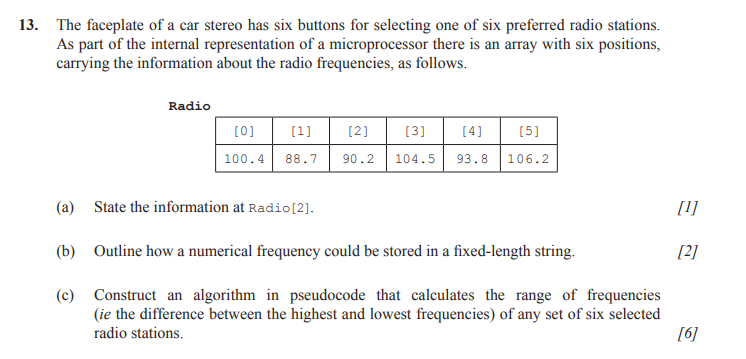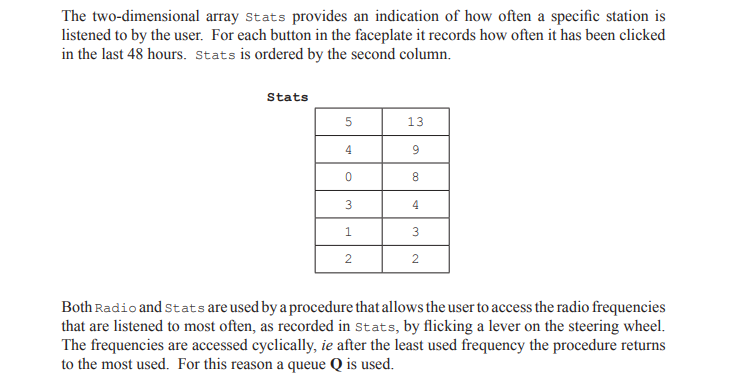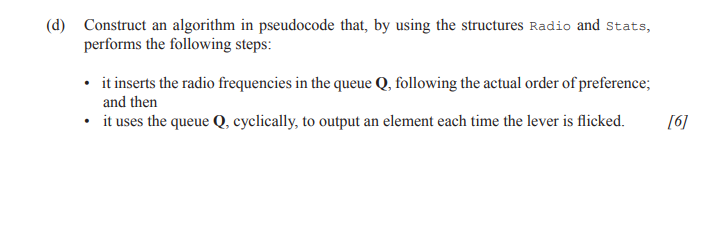## Collections And Trees

A collection — sometimes called a container — is simply an object that groups multiple elements into a single unit. Collections are used to store, retrieve, manipulate, and communicate aggregate data. Typically, they represent data items that form a natural group, such as a poker hand (a collection of cards), a mail folder (a collection of letters), or a telephone directory (a mapping of names to phone numbers).

### Collection methods

Collection methods in Pseudocode are

• .addItem( new data item )
• .resetNext( ) start at beginning of list
• .hasNext( ) checks whether there are still more items in the list
• .getNext( ) retrieve the next item in the list
• .isEmpty( ) check whether the list is empty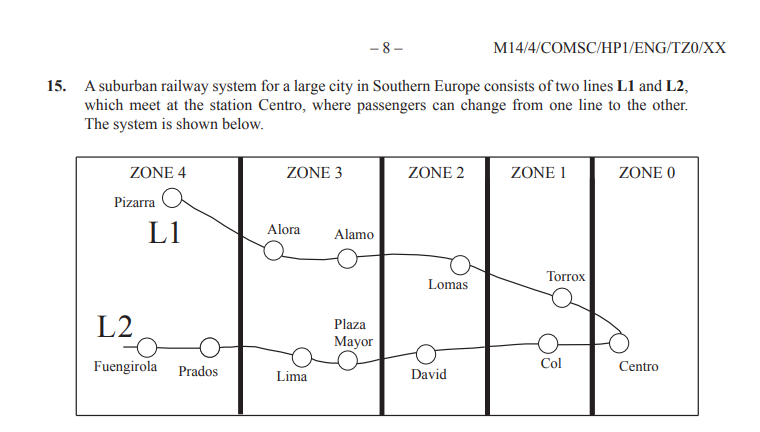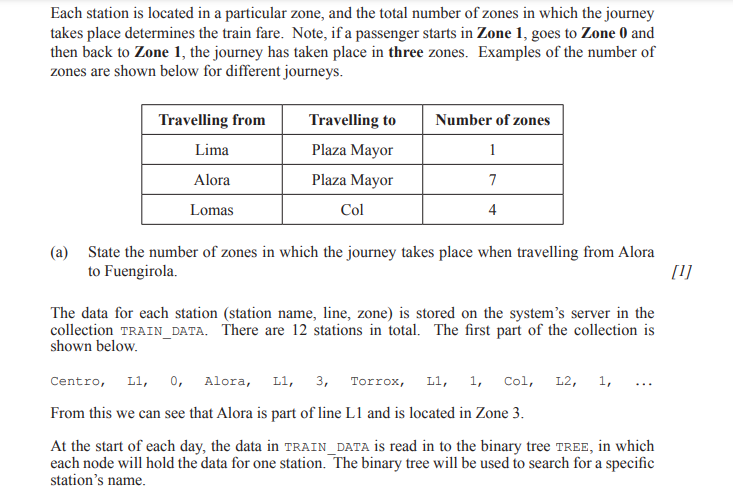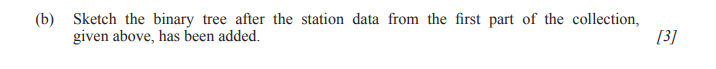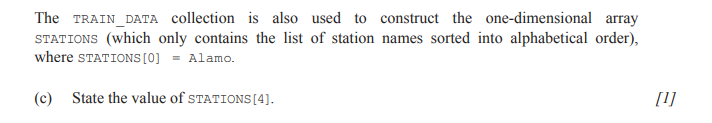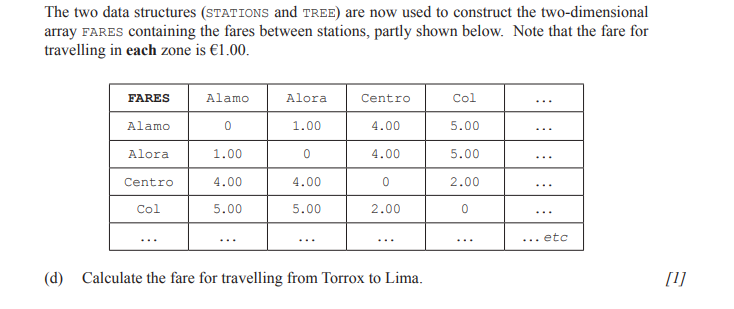# Lesson Block  14 Oct 2021

### 5.1.13 Sketch linked lists (single, double and circular).

Review and Starter / Share

Main/Core Activity

Solution Overview Moderators

11:15  -   11:40

11:40 -  12:10

12:00-12:20

## Stop and think like a Moderator, How are points are awarded?

### part (i)

Spend 5 minutes to answer part (1) below. The marks awarded are 3 points, if you were the IB moderator how would you decide to award the 3 points, you need to be specific. Example award 1 point if student ...........

Review student response and share actual markings scheme.

### part (ii)

Do same for part (ii)### Core Activity

Review Question, but leave for students to complete.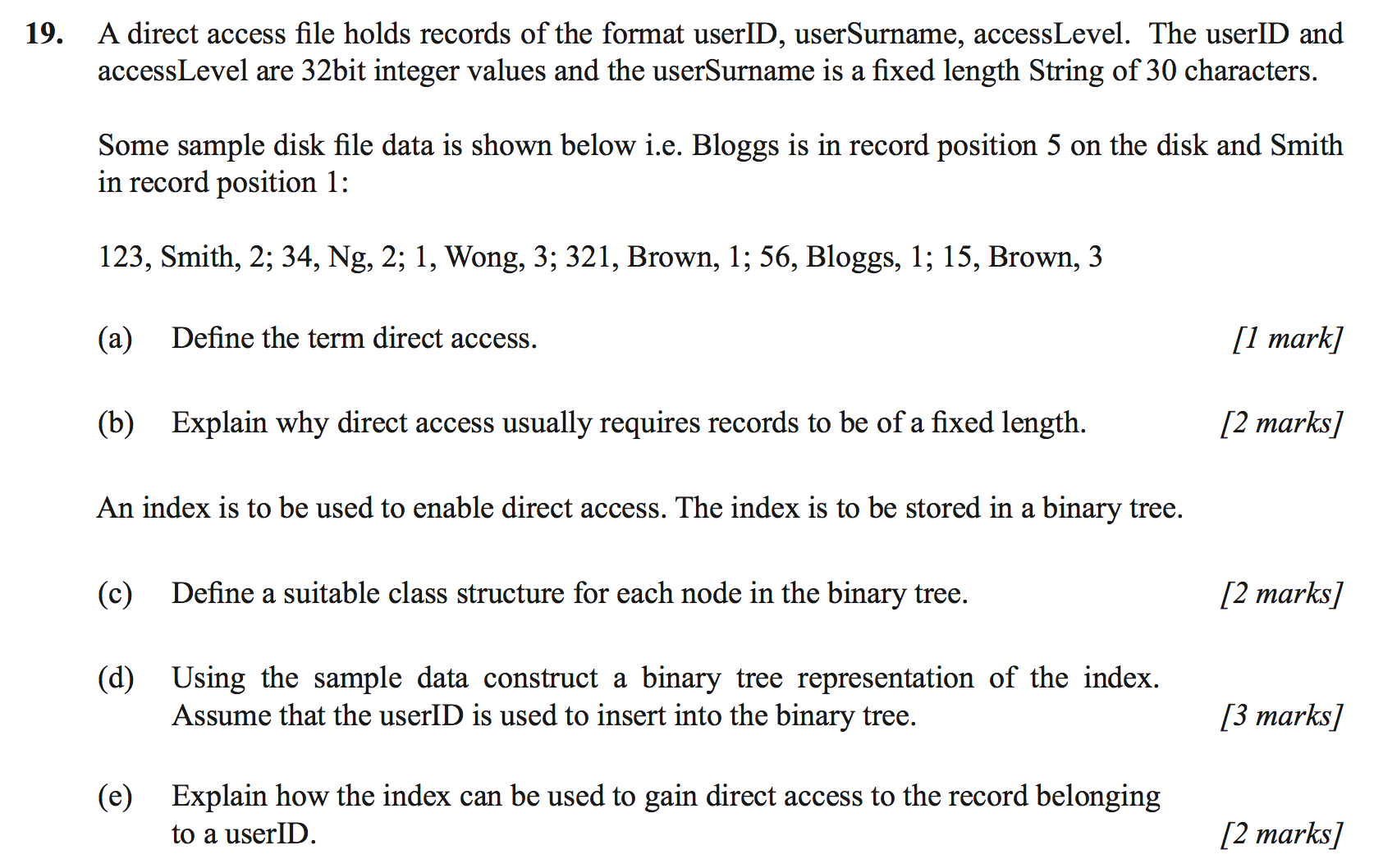# Lesson Block  08 Nov 2021 Review online

4.2.1 Describe the characteristics of standard algorithms on linear arrays. 2 These are: sequential search, binary search, bubble sort, selection sort.

4.2.3 Discuss an algorithm to solve a specific problem. 3 Students should be expected to discuss the differences between algorithms, including both standard and novel algorithms. For example, discussing the advantages and disadvantages of using a binary search as opposed to a sequential search.

# Lesson Block  Bringing It All Together

An election is held in school to elect a president for the student council. There are five candidates, each one represented by a number from

1 – 5. Their names are stored in a corresponding array NAMES.

The students vote electronically and the numbers are entered into the collection VOTES. If any candidate gets more than 50% of the votes, that person is elected, otherwise there is a second vote between the two candidates with the highest number of votes.

Write an algorithm in pseudo code to do the following: